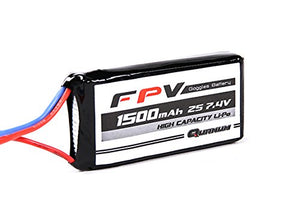# Quanum Fpv Headset Battery 7.4V 1500Mah 3C

HobbyKing

• \$24.64
Unit price per
• Save \$22.69

• B

• O

• O

• O

• L

• E

• I

• N

• C

• .

• r

• e

• s

• e

• a

• r

• c

• h

• e

• s

• a

• n

• d

• d

• e

• v

• e

• l

• o

• p

• s

• e

• l

• e

• c

• t

• r

• o

• n

• i

• c

• p

• r

• o

• d

• u

• c

• t

• s

• a

• n

• d

• o

• p

• e

• n

• s

• o

• u

• r

• c

• e

• h

• a

• r

• d

• w

• a

• r

• e

• w

• i

• t

• h

• t

• h

• e

• a

• i

• m

• o

• f

• p

• r

• o

• m

• o

• t

• i

• n

• g

• M

• A

• K

• E

• R

• S

• P

• A

• C

• E

• a

• n

• d

• D

• I

• Y

• p

• r

• a

• c

• t

• i

• c

• e

• .

• I

• t

• m

• a

• k

• e

• s

• y

• o

• u

• b

• e

• l

• i

• e

• v

• e

• i

• n

• n

• o

• v

• a

• t

• i

• o

• n

• a

• n

• d

• i

• n

• v

• e

• n

• t

• i

• o

• n

• a

• r

• e

• n

• o

• m

• o

• r

• e

• e

• x

• c

• l

• u

• s

• i

• v

• e

• t

• o

• s

• c

• i

• e

• n

• t

• i

• f

• i

• c

• r

• e

• s

• e

• a

• r

• c

• h

• i

• n

• s

• t

• i

• t

• u

• t

• e

• s

• .

Bump up the endurance of your Quanum, Fatshark, or any other set of 5.5mm socket equipped FPV goggles with this 7.4v Lipoly battery with a market-leading 1500 mAh capacity.Double the capacity of the standard pack supplied with most goggles for a modest 15g weight increase!With a handy self-adhesive clip supplied for convenient headstrap mounting, standard charge/discharge and balance leads, this high capacity battery is a must for serious FPV'ers.Specs:Voltage: 7.4vCapacity: 1500mahDimensions: 66 x32 x 13mmWeight: 60gRequired:1 x Fatshark compatible charge adapter such as this item* Suits Fatshark, Boscam, Skyzone aswell as many other types of goggles